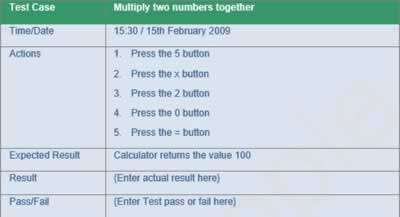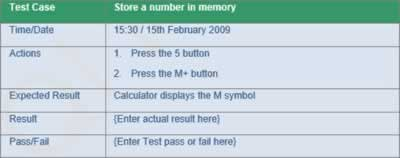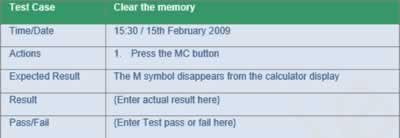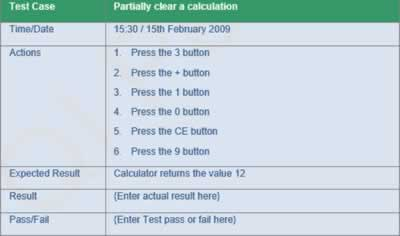# UAT Training

## Calculator User Acceptance Test Cases part 2

(iv) Multiply two numbers together

A UAT test case to multiply two numbers together would be,(v) Store a number in memory

A UAT test case to store a number in memory would be,(vi) Clear the memory

A UAT test case to clear the memory would be,(vii) Partially clear a calculation

A UAT test case to partially clear a calculation would be,